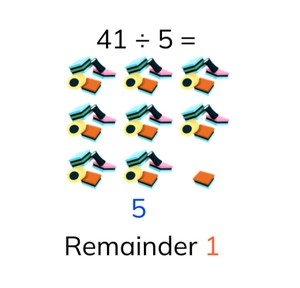Division tables with remainders

# Division tables with remainders

Students learn to solve division tables with remainders.

No account needed.8,000 schools use Gynzy92,000 teachers use Gynzy1,600,000 students use Gynzy

## General

The students learn to solve division problems with remainders using the division tables.

## Standards

CCSS.Math.Content.4.NBT.B.6

## Learning objective

Students will be able to solve the division tables of 1 to 10 with remainders.

## Introduction

The students solve division problems without remainders.

## Instruction

Explain that you first count how many you have of something. You have 19 apples. This is the dividend. Next you count how many you must divide by. You have to divide by 4 children. This is the divisor. The problem is then 19 ÷ 4. Next you see what number in the division table of 4 is the closest to 19. In this case that is 4 × 4 = 16. With 5 × 4 = 20 you have too much. So each child gets 4 apples. Now you subtract the 16 from 19 and have 3 left over. This is the remainder. For the answer you write 4 remainder 3. Next practice the two division problems with remainders together with the students.The students solve the following two problems on their own. Show the problem 49 ÷ 5. Ask the students which table they should look at to find the number closest to 49. Show the table of 5 and explain that 45 is the closest to 49. Next you subtract the 45 from 49. You have 4 left over. Your answer is now 49 ÷ 5 = 9 remainder 4. Have the students solve the problems. Show the table and explain that you are going to divide the number in the column with the number in the top row. The problem is then 28 ÷ 7. Next you repeat the steps to solve the division problem with a remainder. The students solve the problems. In the story problem you first take the numbers from the story that you need to solve the problem (14 ÷ 4). Next you see which number in the table of 4 is the closest to 14. Then you subtract this number to determine the remainder (14 - 12 = 2). Now have the students solve the story problem with the tomatoes.

Check whether the students can solve division problems with remainders using the division tables by asking the following question:
- What steps do you follow to solve the problem 76 ÷ 9?

## Quiz

The students test their understanding of dividing with remainders through ten exercises. Some of the exercises have visual support, others are abstract problems and other exercises are story problems. In one exercise, the students must fill in a division table.

## Closing

Discuss once again the importance of being able to solve a division problem with remainder using the division tables. As a closing activity you can have the students work in pairs to solve the two problems and determine if there is or is not a remainder.

## Teaching tips

Have students that have difficulty solving a division problem with remainder first practice with division problems without remainders. Also have them practice finding in the answer in the table of the divisor. If they understand these concepts, you can have them use concrete materials like pencils or erasers to solve a division problem with a remainder.

## Instruction materials

You may use concrete materials such as pencils or erasers to make dividing with a remainder visual.

### The online teaching platform for interactive whiteboards and displays in schools

• Save time building lessons

• Manage the classroom more efficiently

• Increase student engagement